# How to Build Extruded Maps?

WARNING: this is a work in progress!

## 1 - Principles

the mapextrud R package allows to make extruded maps from sf objects (polygons or multipolygons). For the moment, it is only available on github.

Until a more advanced version is available on CRAN, you can install the package by writing this…

``````library(devtools)
install_github("neocarto/mapextrud")``````

… and load the package like that.

``library(mapextrud)``

## 2 - Basemap setup

The first thing to do before making an extruded map is to deform the basemap to give it a perspective effect. To do this, you can use the deform() function.

We Load an example background map on the US.

``````library(sf)
#> Reading layer `us' from data source `/home/nlambert/Documents/R/mapextrud/inst/us.gpkg' using driver `GPKG'
#> Simple feature collection with 49 features and 3 fields
#> geometry type:  MULTIPOLYGON
#> dimension:      XY
#> bbox:           xmin: -2356114 ymin: -1295495 xmax: 2258154 ymax: 1558935
#> CRS:            unknown``````

See below the original basemap

``plot(st_geometry(us), col="#99aed1", border = "white", lwd=0.4)``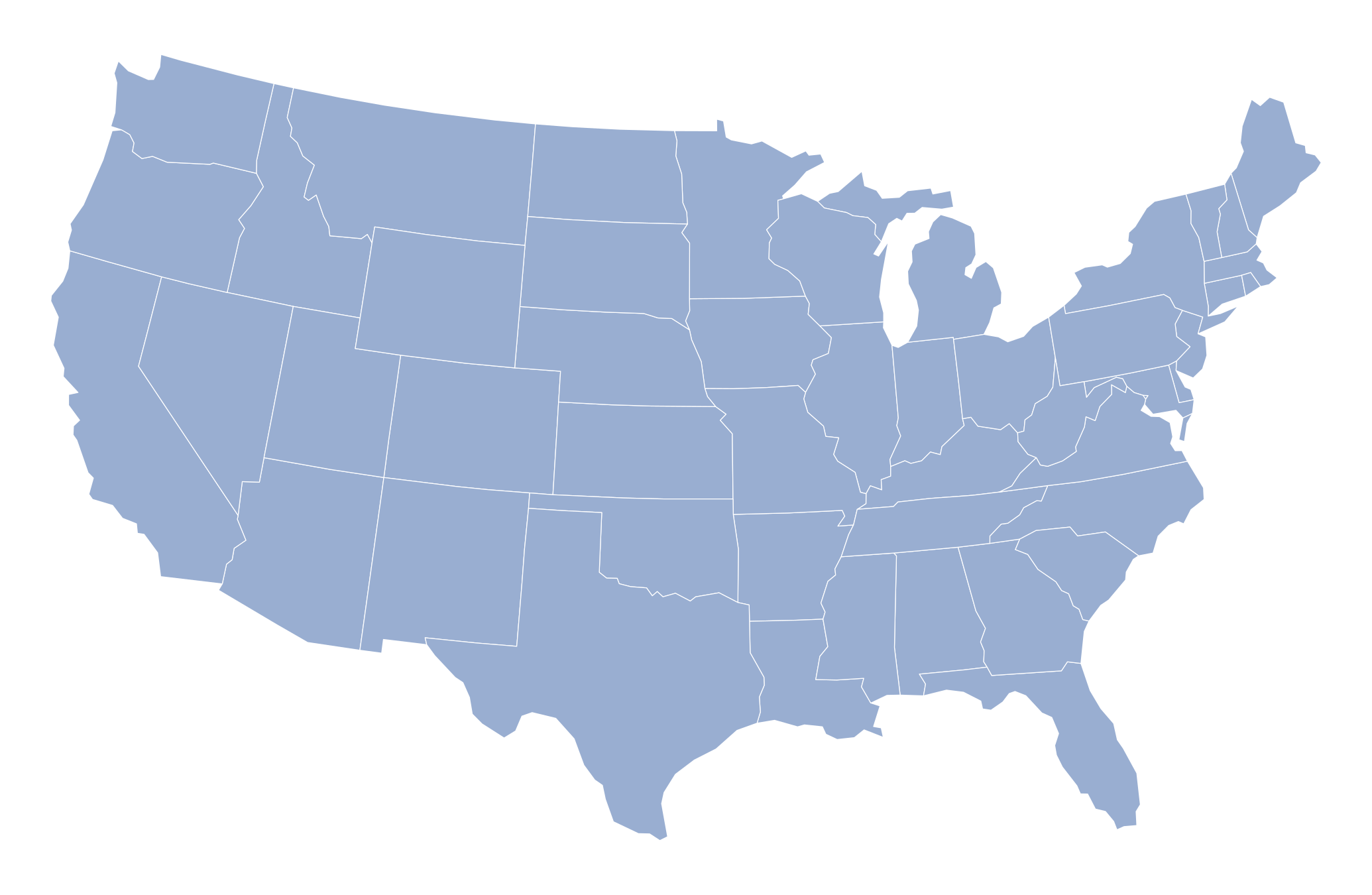Zoom

And here, the deformed basemap.

``````basemap <- deform(us)
plot(st_geometry(basemap), col="#99aed1", border = "white", lwd=0.4)``````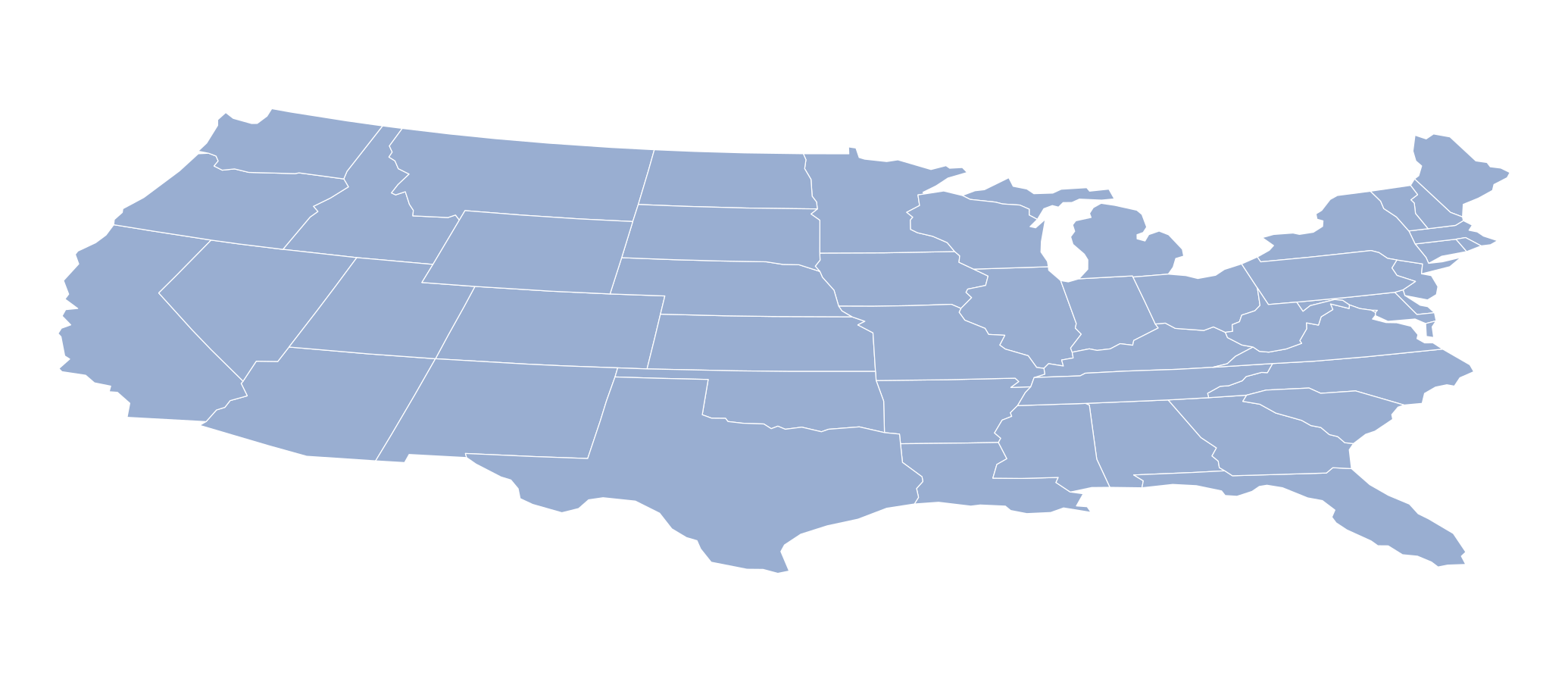Zoom

To better perceive the deformation, you can add a frame with the getframe() function

``````basemap <- deform(us)
frame <- deform(getframe(us))
plot(st_geometry(frame), col="#e1e5eb", border = "#99aed1", lwd=0.4)
plot(st_geometry(basemap), col="#99aed1", border = "white", lwd=0.4, add = TRUE)``````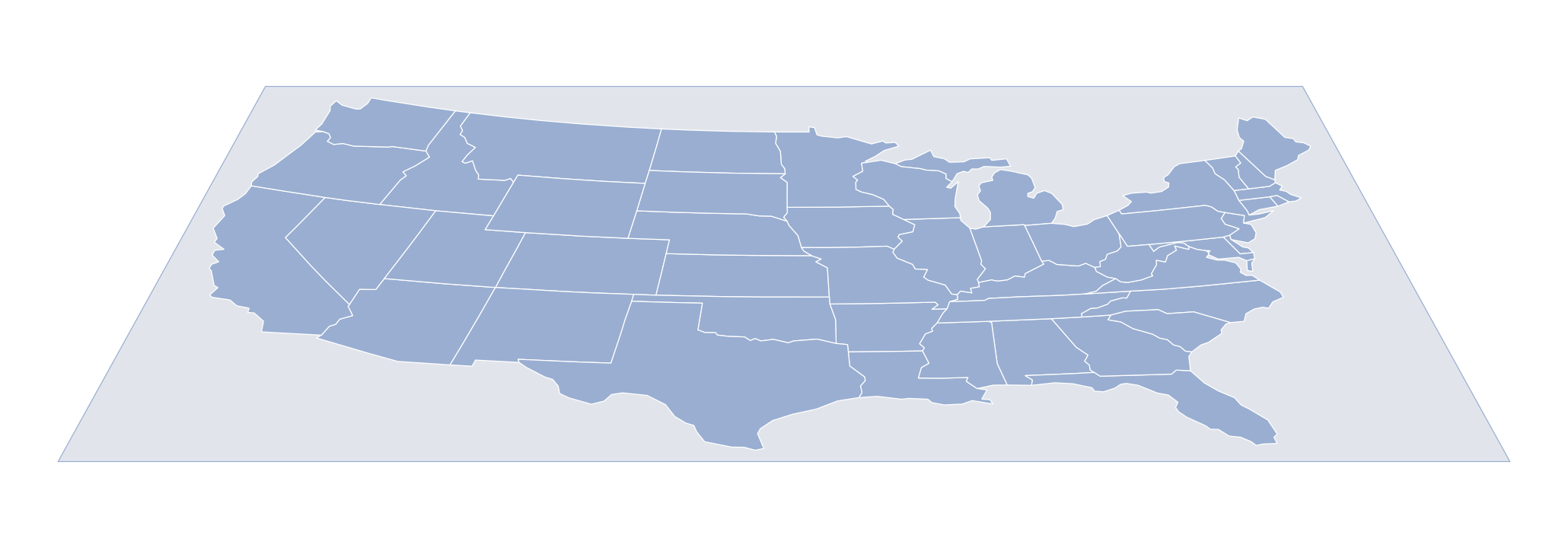Zoom

Notice that you can configure the deformation. The parameter flatten indicates the scaling in Y. The parameter rescale adjust the deformation of the top and bottom of the figure (c(1,1) = no deformation).

``````basemap <- deform(us, flatten=0.5, rescale = c(0.5, 3))
frame <- deform(getframe(us), flatten=0.5, rescale = c(0.5, 3))
plot(st_geometry(frame), col="#e1e5eb", border = "#99aed1", lwd=0.4)
plot(st_geometry(basemap), col="#99aed1", border = "white", lwd=0.4, add = TRUE)``````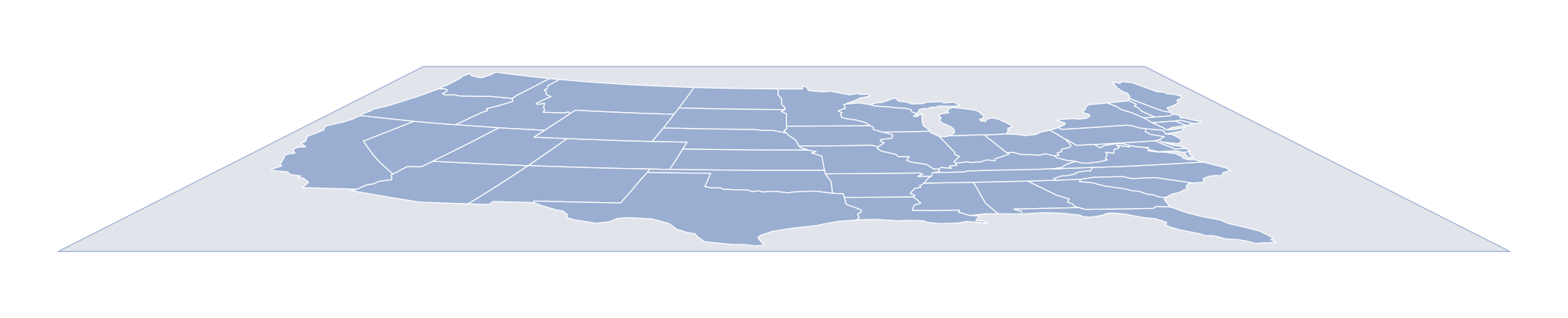Zoom

It can also be useful to rotate the basemap with the rotate() function.

``````us2 <- rotate(us, 180)
basemap <- deform(us2)
frame <- deform(getframe(us2))
plot(st_geometry(frame), col="#e1e5eb", border = "#99aed1", lwd=0.4)
plot(st_geometry(basemap), col="#99aed1", border = "white", lwd=0.4, add = TRUE)``````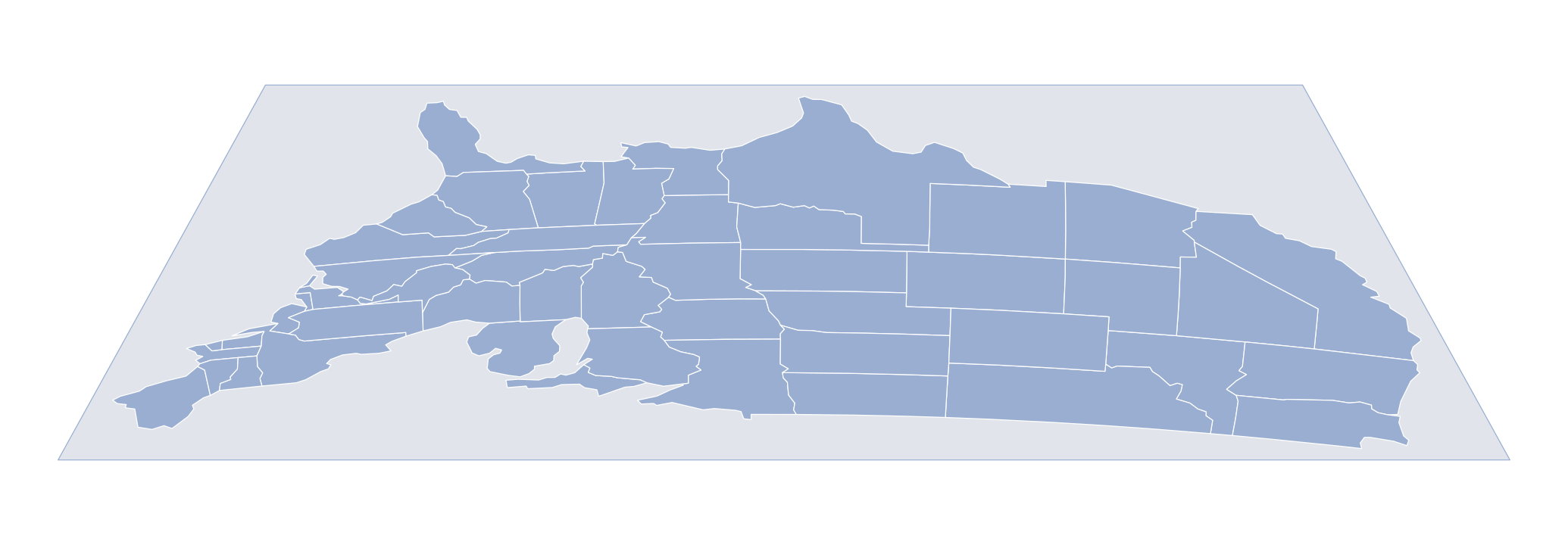Zoom

## 3 - Build an extruded map

Now we’re ready to start extruding… To do this, we use the function - unsustainable suspensions - extrud(). Beware, this is still an experimental function. It has bugs and works very slowly. Sorry for that!

Find below a simple example with the default settings.

``````basemap <- deform(us)
extrude(basemap, var = "pop2019")``````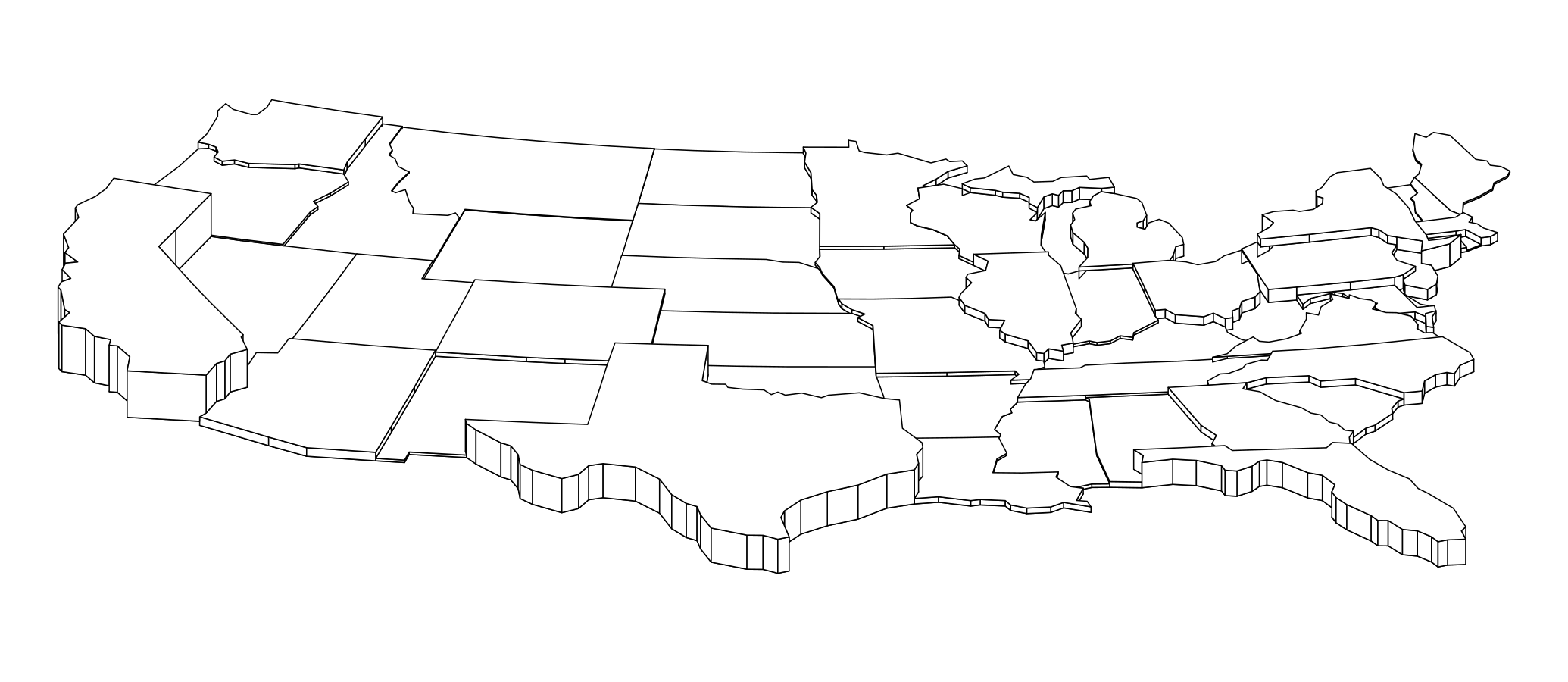Zoom

Of course, you can custom the map by changing colors (col) and heights (k). On the reprentation below, you will probably notice some imperfections. I hope to be able to fix them in the next version of the package.

``````basemap <- deform(us)
extrude(basemap, var = "pop2019", col="#99aed1", k =1.8)``````